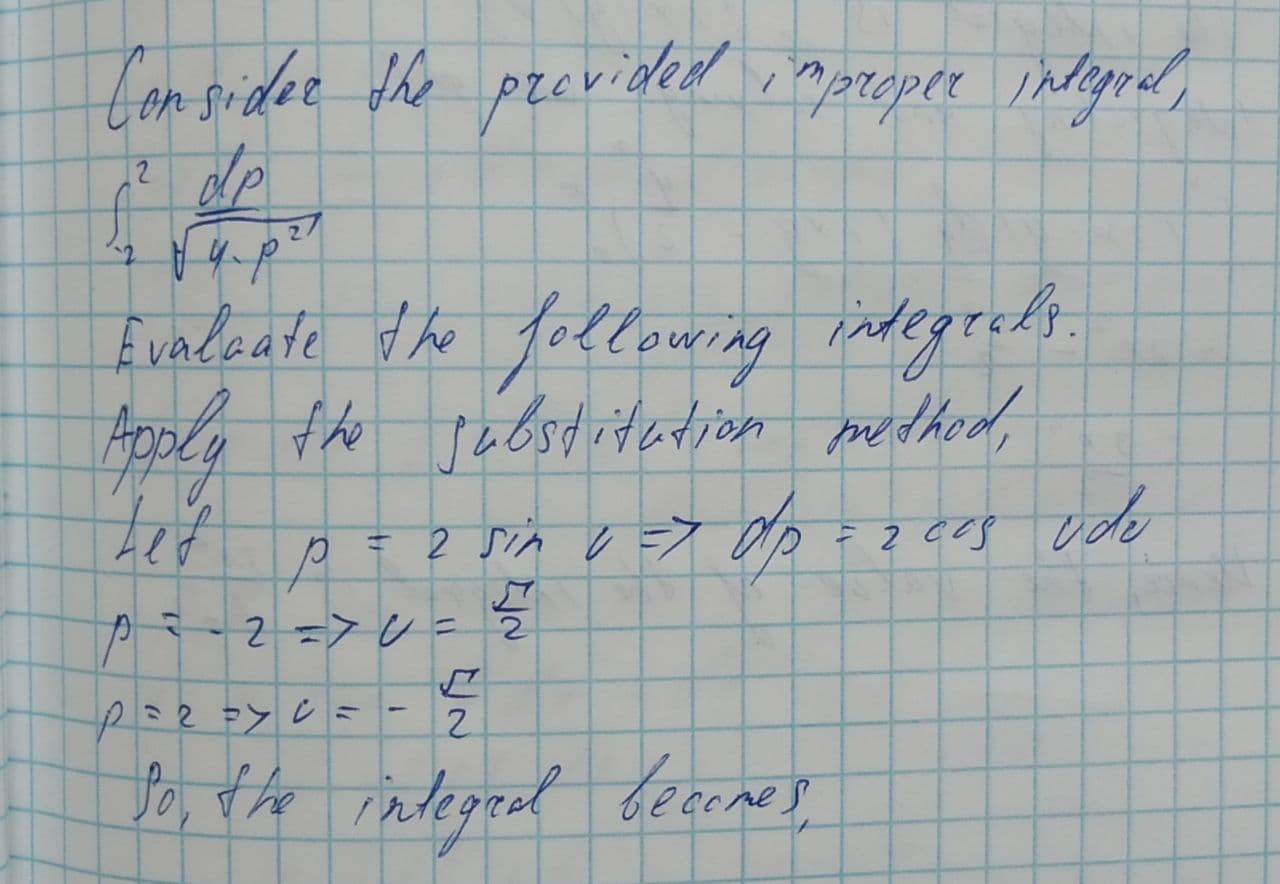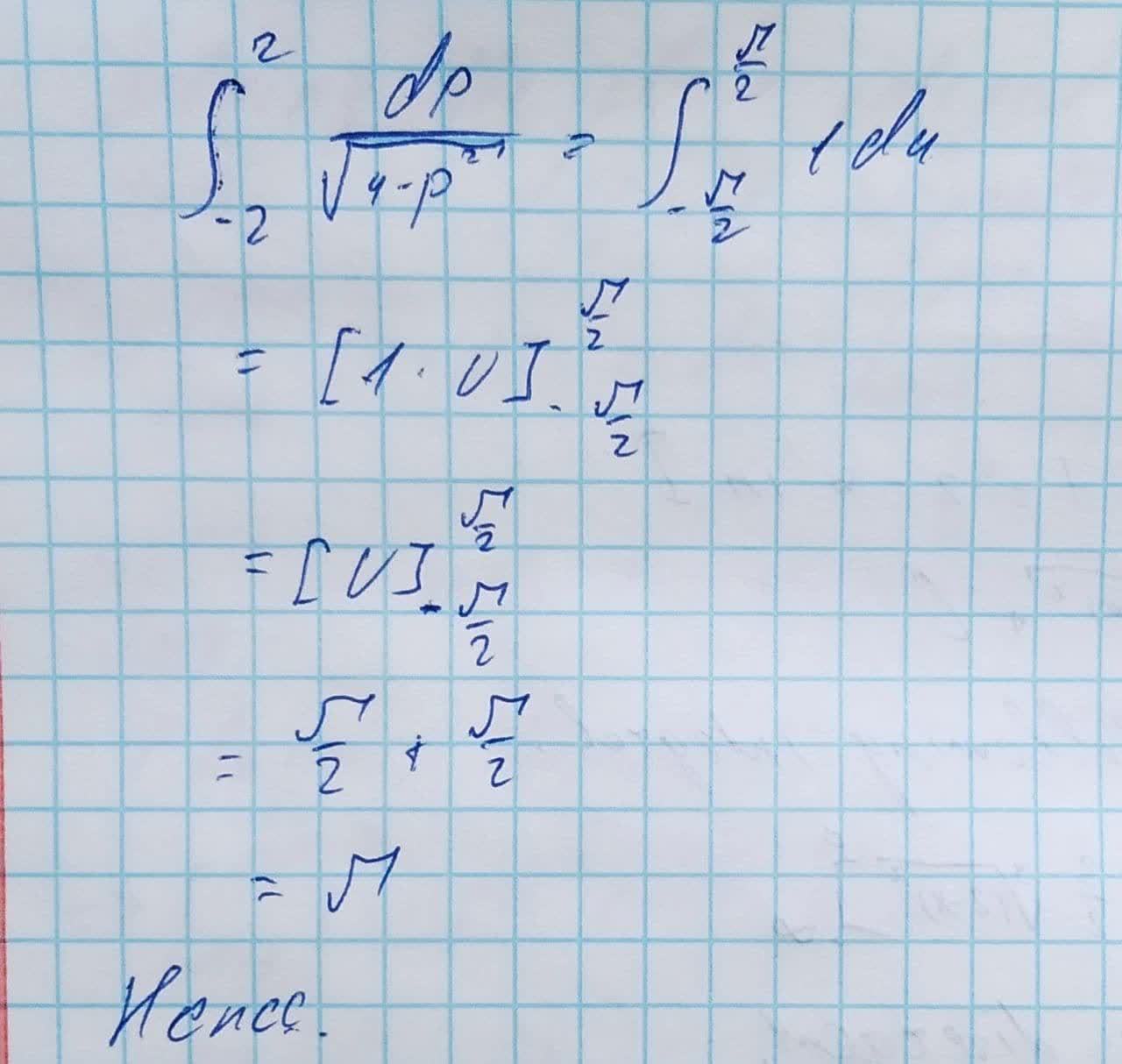Question# Evaluate the following integrals or state that they diverge \int_{-2}{2}\frac{dp}{\sqrt{4-p^{2}}}

Applications of integrals
ANSWEREDEvaluate the following integrals or state that they diverge.
$$\displaystyle\int^{2}_{{-{2}}}{\frac{{{d}{p}}}{{\sqrt{{{4}-{p}^{{{2}}}}}}}}$$